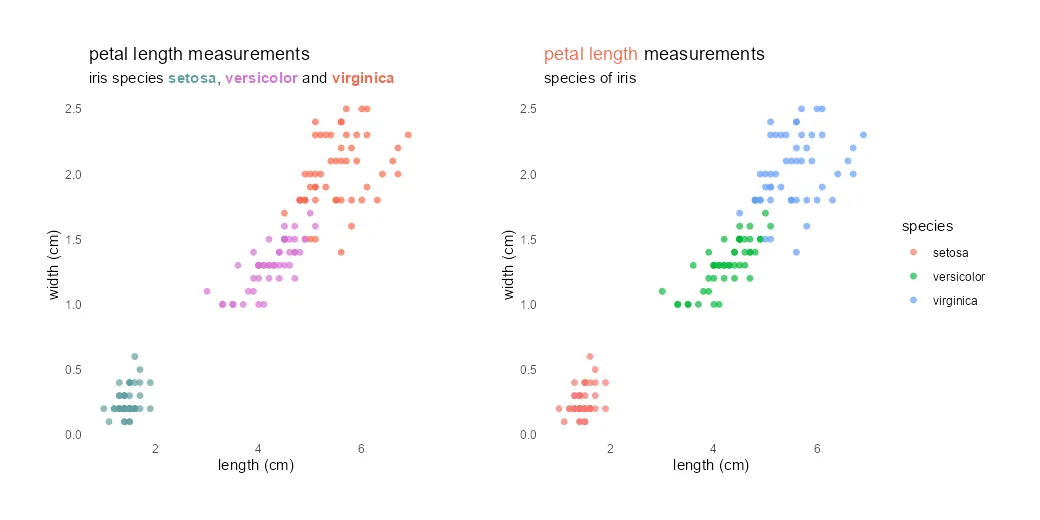# How to use different colors in the ggplot2 title in R

Different colors in the ggplot2 title might be useful to emphasize part of that or as a substitute for the R plot legend. It is not very easy to do, but worth it if it helps to draw the necessary attention.

Here is how my R scatter plot looks at the beginning. That is created with a little formatting effort by removing gridlines and adjusting markers and titles.

```require(ggplot2)

ggplot(iris, aes(x = Petal.Length, y = Petal.Width , color = Species)) +
geom_point(size = 3,
alpha = 0.7,
shape = 16) +
labs(
title = "petal length measurements",
subtitle = "species of iris",
x = "length (cm)",
y = "width (cm)",
color = "species"
) +
theme_minimal() +
theme(panel.grid = element_blank())```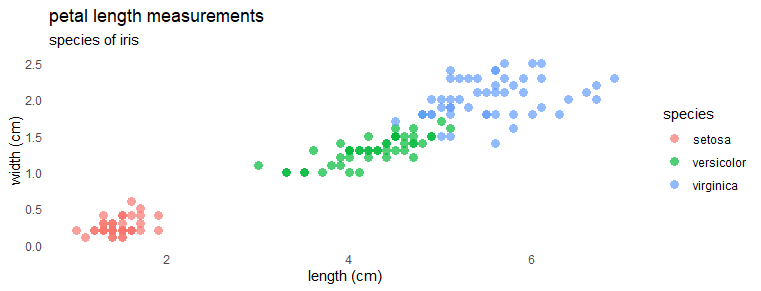## Different colors in the ggplot2 title

By using ggplot2 theme elements, it is possible to change the color of the plot title. Here is what it looks like in a different color.

```ggplot(iris, aes(x = Petal.Length, y = Petal.Width , color = Species)) +
geom_point(size = 3,
alpha = 0.7,
shape = 16) +
labs(
title = "petal length measurements",
subtitle = "species of iris",
x = "length (cm)",
y = "width (cm)",
color = "species"
) +
theme_minimal() +
theme(panel.grid = element_blank(),
plot.title = element_text(color = "slategray"),
plot.subtitle = element_text(color = "slategray"))```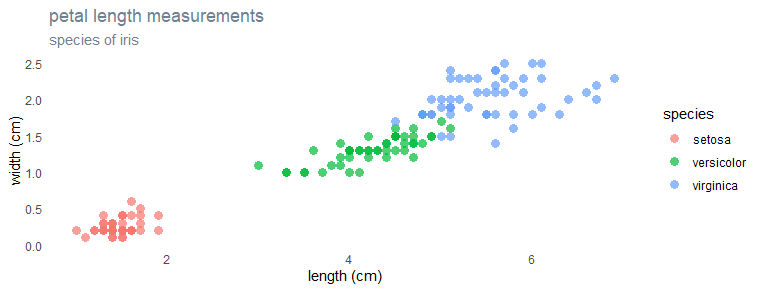To create an R plot with different colors of words in the title, I will be using the ggtext package. It is a ggplot2 extension that provides Markdown and HTML rendering.

```library(ggtext)

ggplot(iris, aes(x = Petal.Length, y = Petal.Width , color = Species)) +
geom_point(size = 3,
alpha = 0.7,
shape = 16) +
labs(
title = "<span style = 'color:tomato;'>petal length</span> measurements",
subtitle = "species of iris",
x = "length (cm)",
y = "width (cm)",
color = "species"
) +
theme_minimal() +
theme(panel.grid = element_blank(),
plot.title = element_markdown())```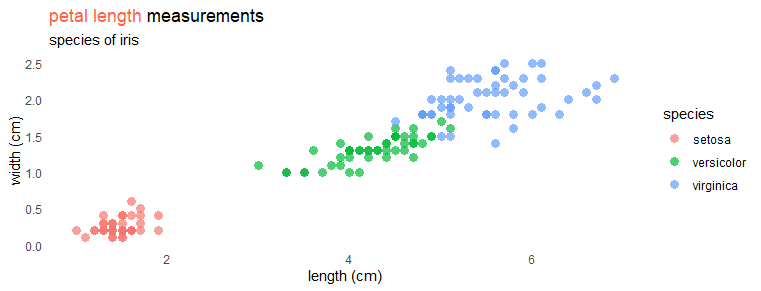Be careful if you are editing existing ggplot2 visualization. Remember to replace element_text with element_markdown to get different colors in the ggplot2 title.

## Substitute ggplot2 plot legend by coloring individual words in the subtitle

Here is another approach to using different colors in the ggplot2 title. In this R plot, the ggplot2 is without legend. Instead, I’m using keywords in the subtitle matching with colors of scatter plot groups. In addition, I used an asterisk symbol to make them appear in bold.

```ggplot(iris, aes(x = Petal.Length, y = Petal.Width , color = Species)) +
geom_point(size = 3,
alpha = 0.7,
shape = 16,
show.legend = FALSE) +
labs(
title = "petal length measurements",
subtitle = "iris species
<span style = 'color:orchid;'>**versicolor**</span> and
<span style = 'color:tomato;'>**virginica**</span>",
x = "length (cm)",
y = "width (cm)",
color = "species"
) +
theme_minimal() +
theme(panel.grid = element_blank(),
plot.subtitle = element_markdown())```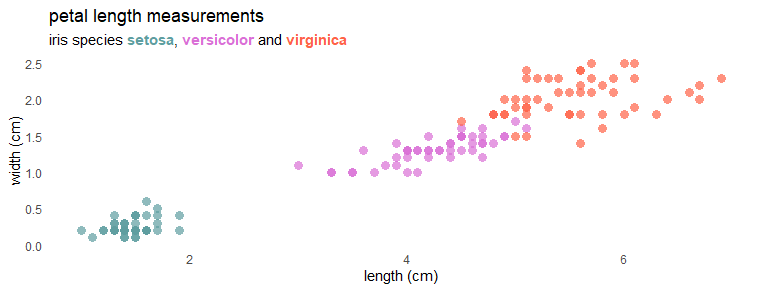I hope it will help you change the color for part of the ggplot2 title, subtitle, or ideas for other enhancements.

Please take a look at other posts from this blog about data visualization or other stuff. For example, how to save R plots or other results temporarily or convert to WebP format.